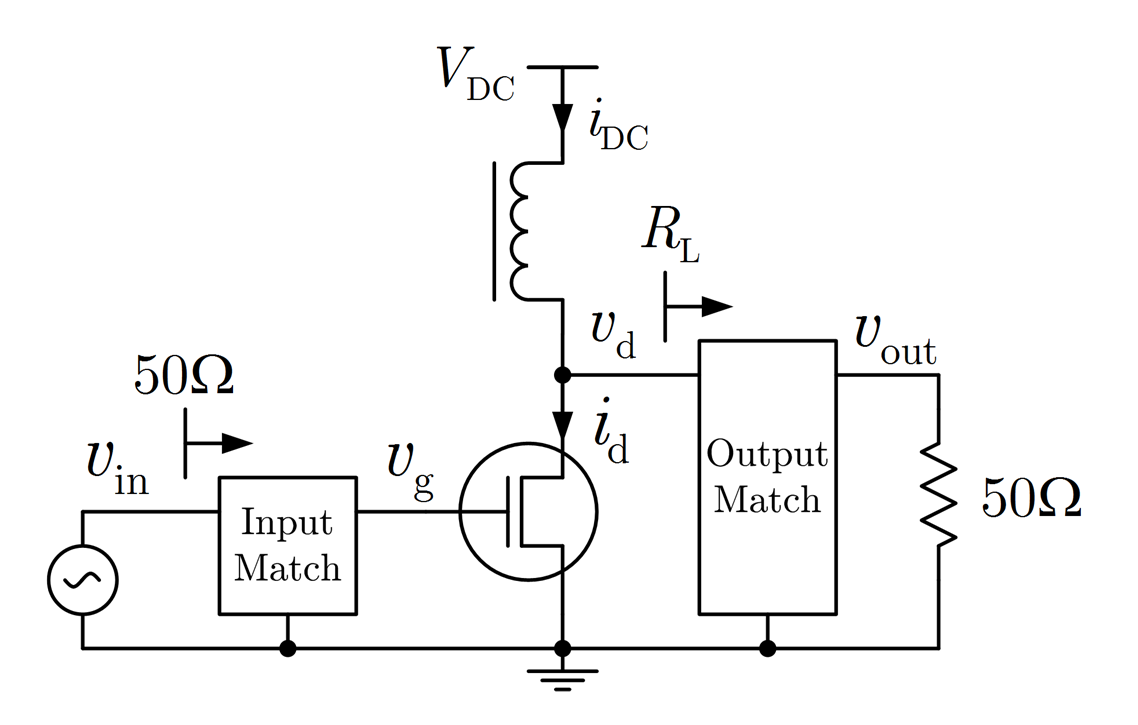Signal Processing and Speech Communication Laboratory

## A Joint Linearity-Efficiency Model of Radio Frequency Power Amplifiers

Published
Mon, Aug 01, 2016
Tags
rotm
ContactWe present an analytical model of the joint linearity-efficiency behavior of radio frequency power amplifiers. The model is derived by Fourier series analysis of a generic amplifier circuit including both strong nonlinearity due to current-clipping as well as weak nonlinearity due to transconductance variation. By selection of the biasing point, common amplifier classes like class A, class B and class AB can be modeled. For numerical evaluation, the model reduces to two lookup-tables, which makes it well suited for high-level system simulations. In an application example we demonstrate how the model can be used to simulate the error-vector-magnitude and the average efficiency for specific single-carrier and multi-carrier modulation schemes.

The figure shows a generic circuit of a radio frequency power amplifier. In the paper we used this circuit as the basis for a joint linearity-efficiency model. A Matlab implementation of the model is available at Matlab Central.

More information can be found in our paper.

Browse the Results of the Month archive.